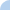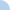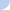# Regression lines and correlation r: session regression lines and correlation r

Use the following: The least-squares regression line based on 43 observations of female white rats is y = 95 + 32x, where x is the weeks after birth and y is weight in grams. The correlation r is 0.78. Directions: Please answer the following questions, and press the submit button when you are done.First name Last nameDavid Farnsworth

 This activity was created by a Quia Web subscriber. Learn more about QuiaCreate your own activities